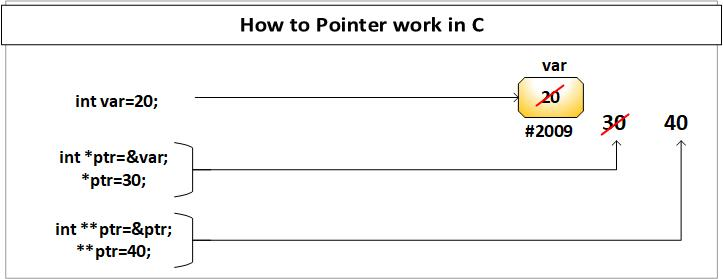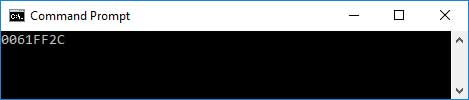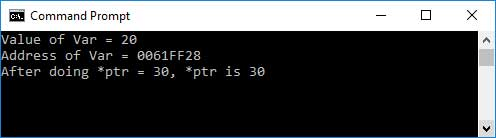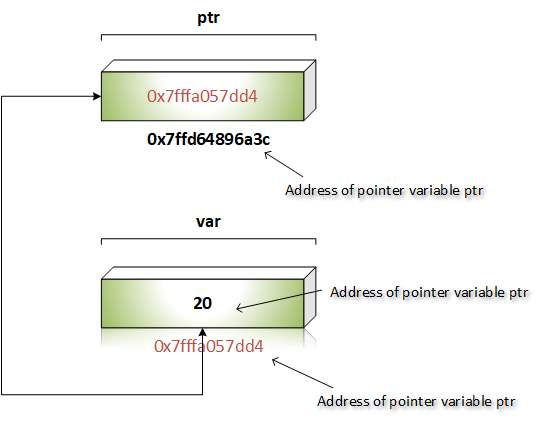# Pointer C Programing

Answer: A pointer is a derived data type in C. Pointers stores memory addresses as their values. Since these memory addresses are locations in computer memory where program instruction and data are stored, pointers can be used to access and manipulate data stored in memory.• Pointers are more efficient in handling arrays and data tables.
• Pointers can be used to return multiple values from a function.
• Pointers permit references to functions and thus allow passing functions as arguments to other functions.
• Using pointer arrays to store character strings, saves data storage space in memory.
• Pointers allow C to support dynamic memory management.
• Pointers reduce length and complexity of programs.
• Pointers increase the execution speed and thus reduce the program execution time.
• Pointers provide an efficient tool for manipulating dynamic data structures such as structure, union, linked list etc.

We use the unary operator & (ampersand) that returns the address of that variable in order to access a variable to a pointer.

For instance & x provides us variable x address.

``````
// The output of this program can be different
// in different runs. Note that the program
// prints address of a variable and a variable
// can be assigned different address in different
// runs.
#include

int main()
{
int x;

printf("%p", &x);

return 0;
}

``````

Output:Declaring the variable pointer: if a variable of pointer in C  is specified, a * must be displayed before the variable name.

``````
// C program to demonstrate declaration of
// pointer variables.
#include
int main()
{
int x = 10;

// 1) Since there is * in declaration, ptr
// becomes a pointer varaible (a variable
// that stores address of another variable)
// 2) Since there is int before *, ptr is
// pointer to an integer type variable
int *ptr;

// & operator before x is used to get address
// of x. The address of x is assigned to ptr.
ptr = &x;

return 0;
}

``````

The unary operator (*) uses the value of the variable that is placed at the address given by its operand to access the value stored in the address.

``````
// C program to demonstrate use of * for pointers in C
#include

int main()
{
// A normal integer variable
int Var = 20;

// A pointer variable that holds address of var.
int *ptr = &Var;

// This line prints value at address stored in ptr.
// Value stored is value of variable "var"
printf("Value of Var = %d\n", *ptr);

// The output of this line may be different in different
// runs even on same machine.
printf("Address of Var = %p\n", ptr);

// We can also use ptr as lvalue (Left hand
// side of assignment)
*ptr = 30; // Value at address is now 30

// This prints 30
printf("After doing *ptr = 30, *ptr is %d\n", *ptr);

return 0;
}

``````

Output:The pictures below of the above program are: